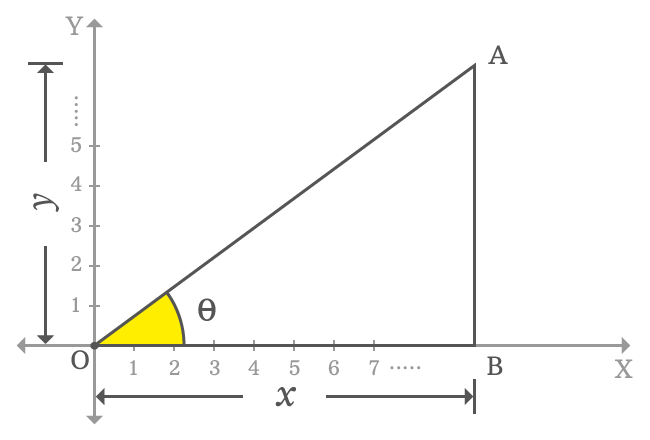# Sign of sin function in first quadrant

## Sign

$\sin{\theta} \, > \, 0$

The sign of value of sin function in first quadrant is always positive.

### Proof

$\Delta BOA$ is a right triangle in first quadrant of the two dimensional Cartesian coordinate system and its angle is taken as theta. The sign of sin function in first quadrant is determined by the ratio of lengths of the opposite side to hypotenuse. So, let’s find the sign of sine function in first quadrant.Actually, the $x$-axis and $y$-axis both represent positive values. Hence, the length of opposite side is denoted by $y$ and the length of adjacent side is denoted by $x$. Therefore, $x > 0$ and $y > 0$.

$\sin{\theta} \,=\, \dfrac{AB}{OA}$

$\implies \sin{\theta} \,=\, \dfrac{y}{\sqrt{x^2+y^2}}$

In this case, the length of opposite side is $y$ and it is positive. So, the value in the numerator is positive. The length of adjacent side is $x$ and it is also positive in first quadrant. Hence, the square root of sum of squares of $x$ and $y$ are positive.

$y > 0$ and $\sqrt{x^2+y^2} > 0$. Therefore, the sign of ratio of them is also positive. Therefore, it is proved that the sign of the value of sin function in first quadrant is positive.

$\, \therefore \,\,\, \sin{\theta} \, > \, 0$

Latest Math Topics
Jun 26, 2023
Jun 23, 2023

###### Math Questions

The math problems with solutions to learn how to solve a problem.

Learn solutions

Practice now

###### Math Videos

The math videos tutorials with visual graphics to learn every concept.

Watch now

###### Subscribe us

Get the latest math updates from the Math Doubts by subscribing us.